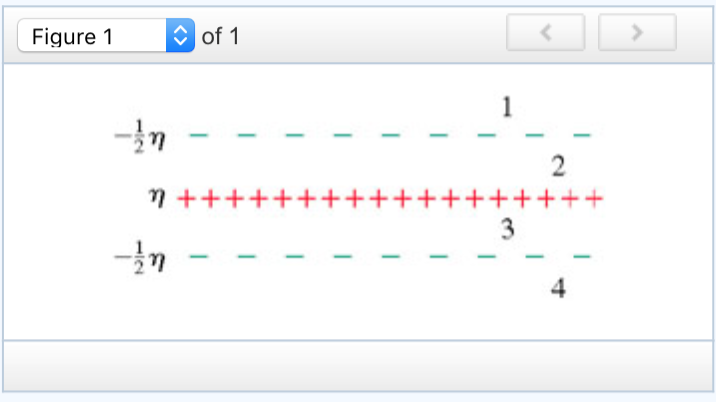# Problem: The three parallel planes of charge shown in the figure (Figure 1) have surface charge densities -1/2 η, η and -1/2 ηPART A ) Find the magnitude of the electric field in region 1.PART B) What is the direction of the electric field in region 1?a. Upwardb. Downwardc. The field is zeroPART C)Find the magnitude of the electric field in region 2.PART D)What is the direction of the electric field in region 2?a. Upwardb. Downwardc. The field is zeroPART E)Find the magnitude of the electric field in region 3.PART F)What is the direction of the electric field in region 3?a. Upwardb. Downwardc. The field is zeroPART G)Find the magnitude of the electric field in region 4.PART H)What is the direction of the electric field in region 4?a. Upwardb. Downwardc. The field is zero

###### FREE Expert Solution

Electric field due to infinitely charged plates:

$\overline{){\mathbf{E}}{\mathbf{=}}\frac{\mathbf{\eta }}{\mathbf{2}{\mathbf{\epsilon }}_{\mathbf{0}}}}$

The electric field lines are normal to the plates (i.e. they point up/down). We'll consider upward to be positive.

Electric field points outwards from a positively charged surface and inward at a negatively charged surface.

90% (286 ratings)###### Problem Details

The three parallel planes of charge shown in the figure (Figure 1) have surface charge densities -1/2 η, η and -1/2 ηPART A ) Find the magnitude of the electric field in region 1.

PART B) What is the direction of the electric field in region 1?
a. Upward
b. Downward
c. The field is zero
PART C)Find the magnitude of the electric field in region 2.

PART D)What is the direction of the electric field in region 2?
a. Upward
b. Downward
c. The field is zero

PART E)Find the magnitude of the electric field in region 3.

PART F)What is the direction of the electric field in region 3?
a. Upward
b. Downward
c. The field is zero

PART G)Find the magnitude of the electric field in region 4.

PART H)What is the direction of the electric field in region 4?
a. Upward
b. Downward
c. The field is zero